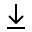MathBin Diophantine Equation - Coins in a Jar Member

This bin is based on the November 17, 2019 column of Ask Marilyn by Marilyn vos Savant, where Marilyn responds to a question posed by Leda Metro of Darien, Conn.

In a contest, a friend guessed the exact number of coins in a jar: 1,692. She won all of the coins, which totaled \$100.54. They consisted of pennies, nickels, dimes and quarters. How many coins of each kind where in the jar? Marilyn asks the reader to start with a guess, and reallocate the coins until they sum to the requested total. More than one answer is possible, and the two Marilyn provides are given below.
QuartersDimesNickelsPenniesTotalGoal
Count
Value (\$)
Sum

### Analysis

This problem gives two linear equations in four unknowns, so the variables cannot be found using straight-up algebra. Using the variables q, d, n, and p to represent quarters, dimes, nickels, and pennies, we have the following two equations. Note that I am using cents instead of dollars in order to use integers instead of decimal numbers.

1. q + d + n + p = 1692
2. 25q + 10d + 5n + p = 10054

It's clear that at least 4 pennies are required, but what is the maximum number of pennies? Having all pennies (p = 1692) does not come close to the required amount in equation (1). To maximize p, we need to use pennies and quarters (why?). The equation to solve is 25(1692 - p) + p ≥ 10054. This yields p ≤ 1343.583, so p = 1343 and q = 349. Plugging these values into the right-hand-side of equation (1) gives 25(349) + 1343 = 10068. Since the result is a little too big, all this means is the maximum number of quarters is 348, the maximum number of pennies is still 1343, and the extra coin is a dime. To wit, 25(348) + 10(1) + 1343 = 10053, which is almost a solution!

If I solved for p in the first equation, I could eliminate p in the second equation.

1. p = 1692 - q - d - n
2. 24q + 9d + 4n = 8362

It's fairly obvious there are constraints on the variables.

• q + d + n < 1689, because we need at least 4 pennies.
• q < 349, even if d and n are zero in equation (1). I already proved this.
• d < 930, even if q and n are zero in equation (1).
• Both q and d cannot be zero. Why?
• n < 1609 because 1608 nickels and 80 quarters is still less than 8362 in equation (1).
• p < 1344. I already proved this.
Possible Solutions
QuartersDimesNickelsPennies
69518511594
68522508594